Machine Learning is dominated by ANN (Automated Neural Network), it requires a large training data set of labelled data to learn a classification model. When only a small data set is available, the decision tree & its variant random forests dominated the classification. In this article, we shall explore the Dempster-Shafer Theory as the theoretical basis for classifiers on a small data set, where classification is operated on the principle of combining pieces of evidence.Figure. Images of Iris spieces. We shall attempt to use the petal width, petal height, sepal width, sepal height measurements to perform classification for the classes of Iris Setosa, Iris Versicolour, or Iris Virginica in this article.

Dempster-Shafer Theory [DST][GS76][GS90] is a mathematical theory of evidence, offers an alternative to traditional probabilistic theory for the mathematical representation of uncertainty. The significant innovation of this framework is that it allows for the allocation of a probability mass to sets or intervals as opposed to mutually exclusive singletons. In contrast, Bayesian inference requires some a priori knowledge and is unable to assign a probability to ignorance. D-S is a potentially valuable tool for the evaluation of risk and reliability in engineering applications when it is not possible to obtain a precise measurement from experiments, or when knowledge is obtained from expert elicitation. An important aspect of D-S theory is the combination of evidence obtained from multiple sources and the modelling of conflict between them.

The motivation for selecting D-S theory can be characterized by the following reasons [SF02]:

• The relatively high degree of theoretical development among the nontraditional theories for characterizing uncertainty.
• The relation of Dempster-Shafer theory to traditional probability theory and set theory.
• The large number of examples of applications of Dempster-Shafer theory in engineering in the past.
• The versatility of the Dempster-Shafer theory to represent and combine different types of evidence obtained from multiple sources.

Using our philosophy of learning by doing, we shall take the practical approach to demonstrate how to use Python pyds Dempster-Shafer module. Starting with some experiment with Dempster-Shafer belief functions, then we shall progress into classification on the “Iris Plant Dataset” [IPD] using D-S theory. The result is achieving 96% accuracy, which is comparable to other ML models.

## Python Installation

### Virtual Environment

Using an isolated Python virtual environment will protect you from headaches and disaster of installations. dst (or your choice of name) is the name of the virtual environment, and python=3.5 is the Python version.

conda create -n dst python=3.5


Press y to proceed. This will install the Python version and all the associated anaconda packaged libraries at {path_to_anaconda_location}/envs/crypto

Then activate the virtual environment by,

source activate dst


### pyds Module

pyds is a Python library for performing calculations in the Dempster-Shafer theory of evidence. This is the best and most comprehensive in the following aspects,

• Support for normalized as well as unnormalized belief functions
• Different Monte-Carlo algorithms for combining belief functions
• Various methods related to the generalized Bayesian theorem
• Measures of uncertainty
• Methods for constructing belief functions from data

Ensure that you are in the virtualenv,

pip install pyds


or it is as easy to install from the downloaded source https://github.com/reineking/pyds

cd pyds; python setup.py install


### Other Modules

The other modules are required to run our experiments,

pip install numpy
pip install pandas
pip install matplotlib
pip install seaborn


These modules are important but not described in details because of this article focus on D-S theory. We shall just use them as processing and displaying tools.

## Dempster-Shafer Evidence Theory

When an expert made an observation on some evidence, he could say,

Expert: “I’m fairly sure that the evidence was either $a$ or $b$. Probably $a$, though it could have been $b$. I could be wrong though.”

The statement about “Probably $a$ or $b$” can be translated into $A = \{a, b\}$, one abstains from making any statement about whether $a$ is more probable than $b$. Instead, such an assignment expresses complete ignorance about this question. There are several equivalent representations for quantifying belief within the D-S belief function framework. The four most important representations are [RT14],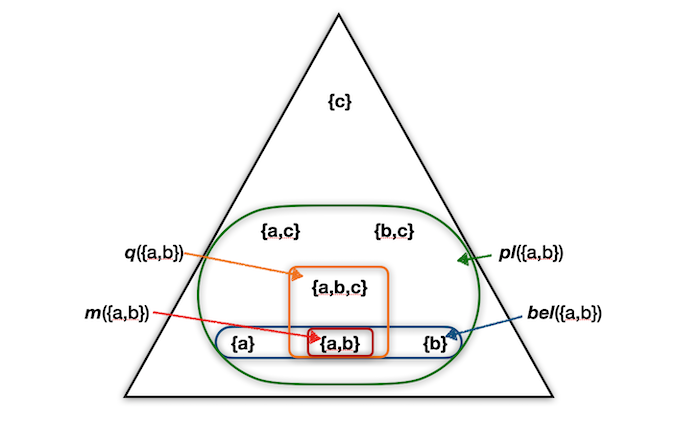Figure. Illustration of different belief function representations. The frame of discernment $\Theta$ consist of three elements $a, b, c$ in this examples. The triangle contains all subsets of $\Theta$ except for $\emptyset$. The indicated sets correspond to the mass of the different belief representations $m, bel, pl,$ and $q$ associated with the set $\{a,b\}$.

Assume the frame of discernment is $\Theta$, and all of possible $\Theta$ subset is U (power set of $\Theta$).

• Mass functions denoted by m: A mass function (also called basic belief assignment or basic probability assignment) is in many respects the most fundamental belief representation and all other representations can be easily obtained from a mass function. Formally, a mass function $m$ is a mapping $m : P(U) \rightarrow [0,1]$ assigning a mass value to each hypothesis $A$ belongs to $U$ of the frame of discernment $\Theta$.  The value $m(A)$ is the amount of belief strictly committed to hypothesis $A$.

• Belief functions denoted by bel: The total amount of belief committed to a hypothesis $A$ subsetof $U$, including all subsets $B$ subset of $A$, is denoted by $bel(A)$. The function $bel : P(U) \rightarrow [0,1]$ is called a belief function. It can be directly computed from a mass function $m$. A belief function $bel$ is sometimes interpreted as de fining a “lower bound” for an unknown probability function $P$.

• Plausibility functions denoted by pl: The plausibility $pl(A)$ is the amount of belief not strictly committed to the complement $\lnot A$. It therefore expresses how plausible a hypothesis $A$ is, i.e., how much belief mass potentially supports $A$. Whereas $bel$ can be viewed as a lower bound for an unknown probability function $P$. Under a lower and upper probability interpretation, the plausibility $pl$ can be viewed as an “upper bound”.

• Commonality functions denoted by q: The commonality $q(A)$ states how much mass in total is committed to $A$ and all of the supersets $A^s$ with $A$ as its subset. The commonality $q(A)$ therefore expresses how much mass potentially supports the entire set.

Let’s test the various belief functions from the pyds MassFunction,

from pyds import MassFunction, powerset

m1 = MassFunction({'a':0.4, 'b':0.2, 'ab':0.1, 'abc':0.3})
m2 = MassFunction({'b':0.5, 'c':0.2, 'ac':0.3, 'a':0.0})
print("m1:",m1)
print("m1: bpa of {'a','b'}=", m1['ab'])
print("m1: belief of {'a','b'}=", m1.bel('ab'))
print("m1: plausibility of {'a','b'}=", m1.pl('ab'))
print("m1: commonality of {'a','b'}=", m1.q('ab'))
print("m2:",m2)
print("m2: bpa of {'b'}=", m2['b'])
print("m2: belief of {'b'}=", m2.bel('b'))
print("m2: plausibility of {'b'}=", m2.pl('b'))
print("m2: commonality of {'b'}=", m2.q('b'))


The results are,

m1: { {'a'}:0.4; {'c', 'a', 'b'}:0.3; {'b'}:0.2; {'a', 'b'}:0.1 }
m1: bpa of {'a','b'}= 0.1
m1: belief of {'a','b'}= 0.7000000000000001
m1: plausibility of {'a','b'}= 1.0
m1: commonality of {'a','b'}= 0.4
m2: { {'b'}:0.5; {'c', 'a'}:0.3; {'c'}:0.2; {'a'}:0.0 }
m2: bpa of {'b'}= 0.5
m2: belief of {'b'}= 0.5
m2: plausibility of {'b'}= 0.5
m2: commonality of {'b'}= 0.5


You can try to test your understand of all belief functions to check with the calculated values.

Alternatively, the MassFunction can be constructed in many different ways, for example,

m1_1 = MassFunction([(('a',), 0.4), (('b',), 0.2), (('a', 'b'), 0.1), (('a', 'b', 'c'), 0.3)])
if (m1_1==m1): print("m1_1 Equal to m1")
m1_2 = MassFunction([('a', 0.4), ('b', 0.2), ('ab', 0.1), ('abc', 0.3)])
if (m1_2==m1): print("m1_2 Equal to m1")


We can get the MassFunction frame of discernment, and the powerset by,

print("frame of discernment", m1.frame())
print("powerset of frame", list(m1.all()))


The results are,

frame of discernment frozenset({'c', 'a', 'b'})
powerset of frame [frozenset(), frozenset({'c'}), frozenset({'a'}), frozenset({'b'}), frozenset({'c', 'a'}), frozenset({'c', 'b'}), frozenset({'a', 'b'}), frozenset({'c', 'a', 'b'})]


We can see pyds MassFunction is using the frozenset, “frozen set” is just an immutable version of a Python set object. While elements of a set can be modified at any time, elements of the frozen set remain the same after creation. Due to this, frozen sets can be used as keys in Dictionary or as elements of another set. But like sets, it is not ordered (the elements can be set at any index).

## Dempster’s Rule of Combination

In order to solve inference problems, belief functions representing different pieces of evidence need to be combined in a meaningful way. This is why combination rules are a major building block of D-S evidence theory. Typically, each piece of evidence is represented by a separate belief function. Combination rules are then used to successively fuse all these belief functions in order to obtain a belief function representing all available evidence.

Specifically, the combination (called the joint mass) is calculated from the two sets of masses $m_1$ and $m_2$ in the following manner:

• $m_{1,2}(\emptyset) = 0$
• $m_{1,2}(A) = (m_1 \oplus m_2) (A) = \frac 1 {1 - K} \sum_{B \cap C = A \ne \emptyset} m_1(B) m_2(C)$

where

• $K = \sum_{B \cap C = \emptyset} m_1(B) m_2(C)$

$K$ is a measure of the amount of conflict between the two mass sets.

Although we are using pyds, its MassFunction has the built-in combination rules. This is still worthwhile to read the following DempsterRule script helps to bring the combination rules into the spotlight with just 10 lines codes.

from numpy import *

# Do NOT use, just for illustration of the D-S combination rules implementation
def DempsterRule(m1, m2):
## extract the frame of discernment
sets=set(m1.keys()).union(set(m2.keys()))
result=dict.fromkeys(sets,0)
## Combination process
for i in m1.keys():
for j in m2.keys():
if set(str(i)).intersection(set(str(j))) == set(str(i)):
result[i]+=m1[i]*m2[j]
elif set(str(i)).intersection(set(str(j))) == set(str(j)):
result[j]+=m1[i]*m2[j]

## normalize the results
f= sum(list(result.values()))
for i in result.keys():
result[i] /=f
return result

m1 = {'a':0.4, 'b':0.2, 'ab':0.1, 'abc':0.3}
m2 = {'b':0.5, 'c':0.2, 'ac':0.3, 'a':0.0}
print(DempsterRule(m1, m2))}


The result of combination is,

{'ac': 0.15789473684210523, 'c': 0.10526315789473682, 'b': 0.5263157894736842, 'ab': 0.0, 'abc': 0.0, 'a': 0.21052631578947364}


Now, back to using pyds D-S combination rule combine_conjunctive() (also defined as a convenience AND (&) operator),

print("Combine m1 and m2:", m1 & m2)
print("Combine m1 and m2 using sampling:", m1.combine_conjunctive(m2, sample_count=10000))


The result of the combined MassFunction is automatically normalized to be the sum of 1.

Combine m1 and m2: { {'b'}:0.5; {'a'}:0.24999999999999994; {'c', 'a'}:0.14999999999999997; {'c'}:0.09999999999999998 }
Combine m1 and m2 using sampling: { {'b'}:0.5015825420622867; {'a'}:0.2518740629685215; {'c', 'a'}:0.14842578710645304; {'c'}:0.09811760786273886 }


## Dempster-Shafer Classifier

Equipped with the D-S theory knowledge, and the usage of pyds MassFunction, the following sections describe the classification on the “Iris Plant Dataset” [IPD] using Dempster-Shafer theory.

### Iris Plant Dataset

The Iris Plant Dataset [IPD] is another standard benchmark problem of UCI datasets. It is a popular choice for testing with classification. The dataset has 150 data items with the following four numeric attributes; Sepal Length, Sepal Width, Petal Length, and Petal Width (all in cm). The classes are the plant types, namely; Iris Setosa, Iris Versicolour, or Iris Virginica, with each class containing 50 samples.The frame of discernment $\Theta$ for the IPD is {Setosa, Versicolour, Virginica}, and the chosen initial classification step allows for seven possible hypotheses (the power set of $\Theta - \emptyset$):

• {Setosa} = class 1
• {Versicolour} = class 2
• {Virginica} = class 3
• {Setosa, Versicolour}
• {Setosa, Virginica}
• {Versicolour, Virginica}
• {Setosa, Versicolour, Virginica}

Continue to import the popular analytic modules to perform some basic data analysis on IPD,

import matplotlib.pyplot as plt
import seaborn as sns
import numpy as np
import pandas as pd
import matplotlib
matplotlib.use('Agg')
matplotlib.style.use('ggplot')


Load the iris plants dataset data/iris.csv into pandas DataFrame df

data_file = r'data/iris.csv'
print('data shape:', df.shape)
df.sample(10)


Randomly select 10 samples from the IPD DataFrame to get some feeling about the data, notice the Class column is the expected classified Iris class.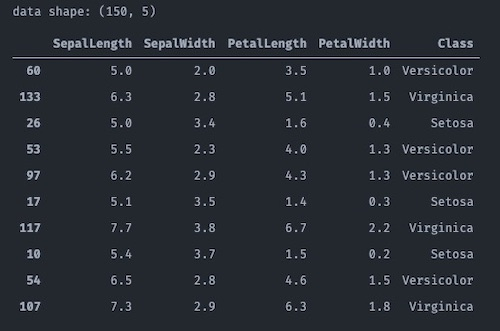For each attribute, we shall plot each class data distribution for further observations.

f, axes = plt.subplots(2, 2, figsize=(12, 10), sharex=True)
for fd, ax in [('SepalLength',axes[0,0]), ('SepalWidth',axes[0,1]), ('PetalLength',axes[1,0]), ('PetalWidth',axes[1,1])]:
for c in df.Class.unique():
sns.distplot(df[df.Class == c][fd], ax=ax, hist=False, label=c, axlabel=fd)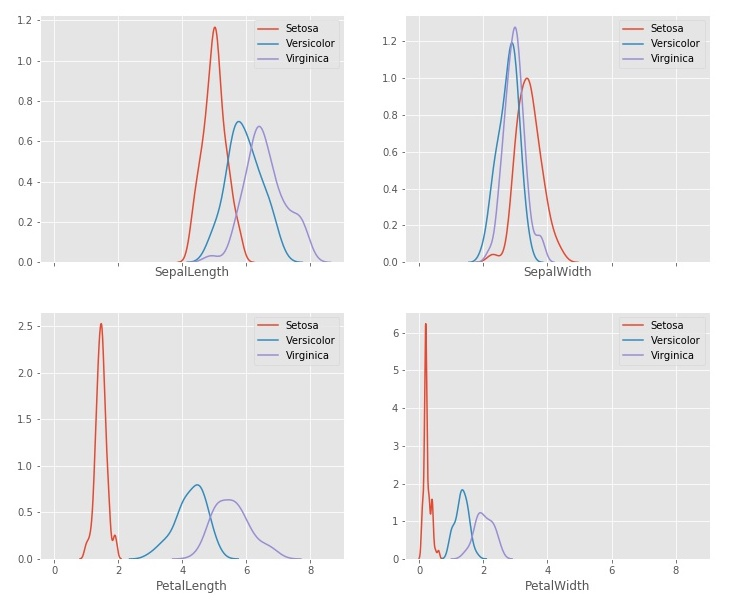From the distribution plots, we can conclude Setosa class is easily separatable by using PetalLength and/or PetalWidth attributes. However, we expected that Versicolor and Virginica classes, will create the most challenges for the classifier.

### Using Dempster-Shafer as Classifier

Following the strategy outlined by [QAUC14], Data Classification Using the Dempster-Shafer Method, the classification can be expressed as a series of steps:

1. Define the frame of discernment ($\Theta$): This is the set of all possible hypotheses related to the given dataset and identifies the classes to which the data must be assigned.
2. Determine class membership: Determine which data attributes are important for establishing class membership and discard the others. In general, the frame of discernment and the selected attributes (their number and their data types) will provide loose guidelines for designing mass functions and the structure of the DRC combinations.
3. Assign mass function: Examine the selected attributes and their data values within a subset of the data in order to design mass functions for each attribute. These functions will be used to assign mass values to the corresponding hypotheses based on the attribute values of the test data.
4. Design DRC strategy: Design a DRC combination strategy based on the data structure. A single application of DRC combines the mass values of each attribute for each data item, but many applications can be used, and DRC can also be used to combine the results of previous applications.
5. Classify data: Following combination, select a rule that converts the result to a decision. Several may be used on different steps, but the final one ultimately classifies the data.

The [QAUC14] does not provide any implementation. We shall follow their research direction to implement a solution in Python to test.

### 1. Define the frame of discernment

The IPD frame of discernment ($\Theta$) is coming from all the unique Class values in the DataFrame df.

pset = dict.fromkeys(powerset(df.Class.unique()), 0)
print(pset)


Here is the power set of the frame of discrenment, where the frozenset() is the empty set.

{frozenset(): 0,
frozenset({'Virginica'}): 0,
frozenset({'Setosa', 'Virginica'}): 0,
frozenset({'Versicolor'}): 0,
frozenset({'Versicolor', 'Virginica'}): 0,
frozenset({'Setosa'}): 0,
frozenset({'Setosa', 'Versicolor'}): 0,
frozenset({'Setosa', 'Versicolor', 'Virginica'}): 0}


### 2. Determine class membership

Since IPD attributes are all numeric data, the easiest way to determine a class membership is by the attribute’s minimum and maximum values. Later, we shall use these attribute data ranges to assign the class membership.

classRange = {}
for c in df.Class.unique():
fieldRange = {}
for f in fields:
fieldRange[f] =(df[df.Class == c][f].min(), df[df.Class == c][f].max())
classRange[c] = fieldRange
print(classRange)


For each attribute, we found the class’s data range of [min, max] values,

{
'Setosa': {
'PetalLength': (1.0, 1.9),
'PetalWidth': (0.1, 0.6),
'SepalLength': (4.3, 5.8),
'SepalWidth': (2.3, 4.4)},
'Versicolor': {
'PetalLength': (3.0, 5.1),
'PetalWidth': (1.0, 1.8),
'SepalLength': (4.9, 7.0),
'SepalWidth': (2.0, 3.4)},
'Virginica': {
'PetalLength': (4.5, 6.9),
'PetalWidth': (1.4, 2.5),
'SepalLength': (4.9, 7.9),
'SepalWidth': (2.2, 3.8)}
}


### 3. Assign mass functions

This step provides a rough method for grouping the data, as can be seen from the diagram, some items may not be classified into a single class. For example, if an attribute value is less than min(class 2), and greater than or equal to min(class 1), the data item must belong to class 1. However, if the value is less than min(class 3), and greater than or equal to min(class 2), the data item could belong to either class 1 or class 2. The process is repeated for each attribute and mass values are assigned in the following way. For data items that are assigned to one class only: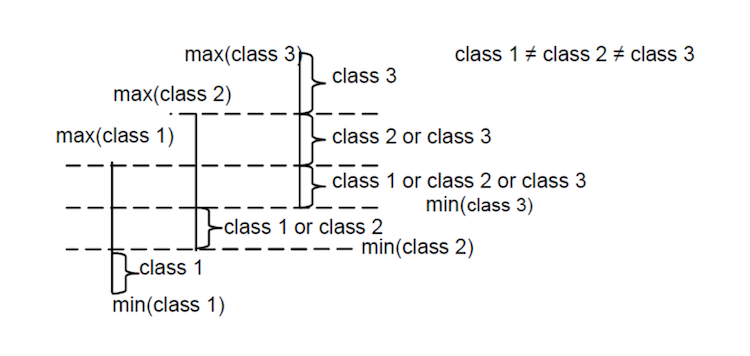credit: picture from [QAUC14]

• $m(\text{class x}) = 0.9, m(\Theta) = 0.1,$

i.e. the uncertainty m($\Theta$) is fixed at 0.1. For data items assigned to two possible classes x and y:

• $m(\text{class x} \cup \text{class y}) = 0.9, m(Θ) = 0.1$

For data items assigned as possibly belonging to all three classes:

• $m(\Theta) = 1$

i.e. the greatest uncertainty where $m(\Theta)$ is 1, zero knowledge of a specific hypothesis.

def hypothesis(df, classRange, fieldName, value):
hset = set()
for c in df.Class.unique():
if (classRange[c][fieldName] <= value and value < classRange[c][fieldName]):
return frozenset(hset)

def hypothesisCounts(hypothesisCount, h, singletons, frame):
if h in singletons:
hypothesisCount[h] += 0.9
hypothesisCount[frame] += 0.1
elif h == frame:
hypothesisCount[frame] += 1.0
else:
hypothesisCount[h] += 0.9
hypothesisCount[frame] += 0.1

hypothesisCount = dict.fromkeys(powerset(df.Class.unique()), 0)
singletons = MassFunction(hypothesisCount).singletons()
frame = frozenset(df.Class.unique())

m = {}
for f in fields:
hypothesisCount = dict.fromkeys(powerset(df.Class.unique()), 0)
for index, row in df.iterrows():
h = hypothesis(df, classRange, f, row[f])
hypothesisCounts(hypothesisCount, h, singletons, frame)
m[f] = MassFunction(hypothesisCount).normalize()
print(f, 'MassFunction =', m[f])


The result MassFunction are,

SepalLength MassFunction = {
{'Versicolor', 'Setosa', 'Virginica'}:0.4446680080482897; {'Versicolor', 'Virginica'}:0.3863179074446678; {'Setosa'}:0.0965794768611671; {'Virginica'}:0.07243460764587531; {'Versicolor'}:0.0; {'Setosa', 'Virginica'}:0.0; {'Versicolor', 'Setosa'}:0.0
}
SepalWidth MassFunction = {
{'Versicolor', 'Setosa', 'Virginica'}:0.7645875251509054; {'Setosa', 'Virginica'}:0.1448692152917505; {'Setosa'}:0.06639839034205235; {'Versicolor', 'Virginica'}:0.018108651911468817; {'Versicolor'}:0.006036217303822939; {'Virginica'}:0.0; {'Versicolor', 'Setosa'}:0.0
}
PetalLength MassFunction = {
{'Setosa'}:0.29327902240325865; {'Virginica'}:0.25050916496945014; {'Versicolor', 'Virginica'}:0.1771894093686355; {'Versicolor'}:0.1771894093686355; {'Versicolor', 'Setosa', 'Virginica'}:0.10183299389002021; {'Setosa', 'Virginica'}:0.0; {'Versicolor', 'Setosa'}:0.0
}
PetalWidth MassFunction = {
{'Setosa'}:0.3012295081967213; {'Virginica'}:0.264344262295082; {'Versicolor'}:0.1721311475409837; {'Versicolor', 'Virginica'}:0.15983606557377059; {'Versicolor', 'Setosa', 'Virginica'}:0.10245901639344246; {'Setosa', 'Virginica'}:0.0; {'Versicolor', 'Setosa'}:0.0
}


### 4. Design DRC strategy

The system combines the mass values from all four attributes using DRC, thus producing overall mass values for all hypotheses, and the hypothesis with the highest belief value is used to classify the data item. If the hypothesis does not represent a single class, then a second step is necessary.

In the second step, for each attribute, standard deviations ($sd$) are calculated for each individual class in question and for the union of those classes. The Feature Selection Value ($FSV$) is then calculated based on:

• $FSV = \frac{sd(class_1)×sd(class_2)×⋅⋅⋅×sd(class_n)}{sd(class_1 \cup class_2 \cup ⋅⋅⋅ \cup class_n)}$

where n is a natural number representing the number of classes. The attribute a that has the smallest $FSV$ is selected, and the absolute difference $d$ (distance) between the data item’s $a$ value and the mean $a$ value is calculated for each class,

• $d_i = |a_i − mean(a_i)|, i = 1,...,n$

The data item is classified as belonging to the class $x$ with the smallest $d$ value.

import functools

def min_fsv_attr(sdf, fields):
fsv = {}
for f in fields:
l = list(sdf.loc[:, f]['std'])
fsv[f] = functools.reduce(lambda a,b: a*b, l) / df[f].std()
return min(fsv.keys(), key=(lambda k: fsv[k]))

def min_dist_attr(sdf, fsv_attr, candidates, sample):
dist = {}
for c in candidates:
dist[c] = abs(sample[fsv_attr] - sdf.loc[c, fsv_attr]['mean'])
return min(dist.keys(), key=(lambda k: dist[k]))

sdf = df.groupby('Class').describe()
fsv_attr = min_fsv_attr(sdf, fields)
print(fsv_attr)


After call the min_fsv_attr, we know the best attribute that has smallest $FSV$ is PetalWidth.

### 5. Classify data

The complete classification workflow is illustrated in a flow chart,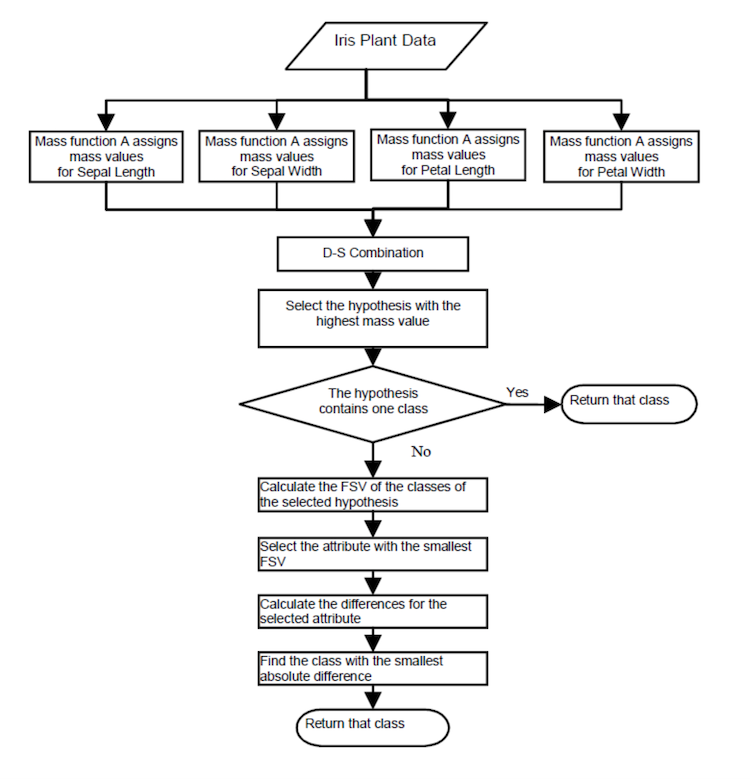credit: picture from [QAUC14]

Combining with the former code fragments and the following code, the D-S Classifier implementation is completed.

def predict(df, combine):
best = 0.0
bestClass = frozenset({})
for c in combine.all():
if (combine[c] > best):
best = combine[c]
bestClass = c
return (bestClass, best)

countFail = 0
countPredict = 0
ms = {}
for index, row in df.iterrows():
sample = row
for f in fields:
hypothesisCount = dict.fromkeys(powerset(df.Class.unique()), 0)
h = hypothesis(df, classRange, f, sample[f])
hypothesisCounts(hypothesisCount, h, singletons, frame)
ms[f] = MassFunction(hypothesisCount).normalize()

# which is doing - ms['SepalLength'] & ms['SepalWidth'] & ms['PetalLength'] & ms['PetalWidth']
combine_mf = functools.reduce(lambda a,b: a & b, ms.values())

(bestClass, best) = predict(df, combine_mf)
if (bestClass in singletons) and (best > 0.8):
# if a singleton class and the confidence is greater than 80%
countPredict += 1
if bestClass != frozenset({sample.Class}):
# print('failed predict:', set(bestClass), best, 'expect:', set({sample.Class}))
countFail +=1
else:
# not a singleton class, find by second step strategy
# print(set(bestClass), best)
fsvClass = min_dist_attr(sdf, fsv_attr, list(bestClass), sample)
if (best > 0.8):
countPredict += 1
if fsvClass != sample.Class:
# print('FSV failed predict:', set({fsvClass}), best, 'expect:', set({sample.Class}))
countFail +=1

print('total rows', df.Class.count())
print('total predicted', countPredict)
print('total fail predicted', countFail)
print('accuracy rate', (countPredict - countFail)/(countPredict*1.0))


The reported prediction accuracy is 96%!

total rows 150
total predicted 150
total fail predicted 6
accuracy rate 0.96


The results suggested that this particular D-S classifier is equivalent to other leading approaches in its ability to classify items with multiple classes and fewer attributes.

## Concluding Remarks

Dempster-Shafer based classifiers have the flexibility to be designed in solving any given problem. In particular, we can decide how the mass functions should be constructed or how the data combination should be organized. One can build mass functions that are as simple or as complex as desired, and Dempster’s rule of Combination can be applied many times using different strategies. This is a distinct advantage in that it allows the creation of systems tailored towards a given problem domain, but it may be argued that it is also a disadvantage, since it does not permit the generalization of results to all domains.

Both theoretical and practical advantages of D-S has been reiterated in many D-S applications. D-S does not require a priori knowledge (data labels), making it suitable for unsupervised classification - possibly classifying previously unseen information, for example, if the aim is to detect previously unseen network attacks in computer systems. Furthermore, a value for ignorance can be modelled, providing information on the uncertainty of an observation.

## References

### Technical References

• [DST] Dampster-Shafer Theory Wikipedia
• [GS76] Glenn Shafter, A Mathematical Theory of Evidence, 1976, Princeton University Press, Princeton, N.J
• [IPD] Iris Plant Dataset, UCI Machine Learning Repository
• [GS90] Jean Gordon and Edward H. Shortliffe, Chapter 13: The Dempster-Shafer theory of evidence, Rule-Based Expert Systems: The MYCIN Experiments of the Stanford Heuristic Programming Project, June 1990, isbn:9780201101720
• [PYDS] Python Dempster-Shafer pyds` module
• [QAUC14] Qi Chena, Amanda Whitbrooka, Uwe Aickelina, Chris Roadknightab, Data Classification Using the Dempster-Shafer Method, (2014) Data classification using the Dempster-Shafer method. J Exper Theor Artif Intell 26:493–517.
• [SF02] Karl Sentz and Scott Ferson, Combination of Evidence in Dempster-Shafer Theory, Sandia National Laboratories Report, April 2002
• [RT14] Thomas Reineking, Belief Functions: Theory and Algorithms, Thesis, Feb 2014.